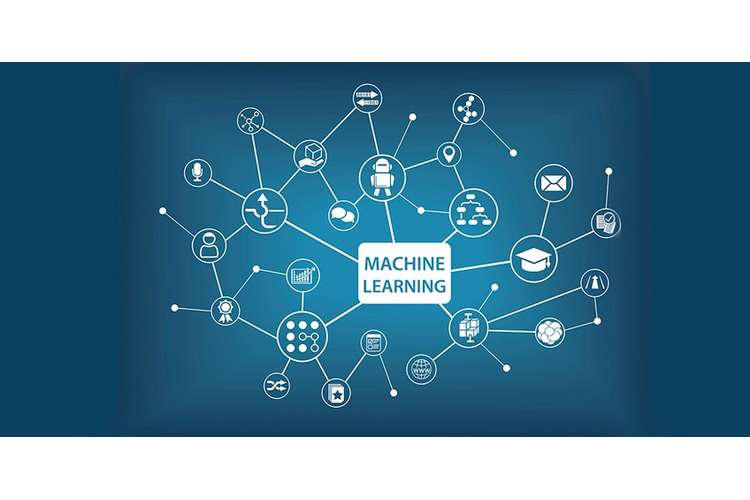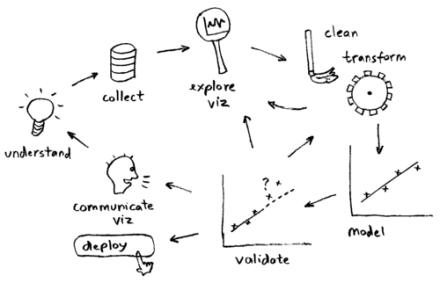Machine Learning Proof Reading and Editing ServicesMachine Learning Proof Reading and Editing Services

This assignment explains about the application of artificial intelligence that provides the ability to learn and improve from experience automatically without explicitly programmed

Assessment 2

This assignment has 25% value

The length of the assignment should be 30 pages maximum

Learning Outcomes

This assessment assesses the following Learning outcomes:

1. Unit Learning Outcomes

a. Work collaboratively and apply linear and logistic regression, and linear Support Vector Machines for designing accurate classifier.

b. Implement model selection and compute relevant evaluation measure for a given problem.

a.Discipline capabilities and knowledge

b. Critical thinking

c. Problem Solving

Objective

In this assessment, students have to demonstrate their skills for applying regularized logistic regression and to perform two-class and multi-class classification for real-world tasks. Also, they have to demonstrate their skill in recognizing under-fitting/over fitting situations.

Instructions

1. Students will be required to analyse a given real-world scenario and contribute to the classifier design.

2. Students will be required to consolidate their individual solutions and propose best solution that for the group’s response to solving the problem.

1. Data Munging (3 Marks)

Cleaning the data is essential when dealing with real world problems. Training and testing data is stored in "data/wisconsin_data" folder. You have to perform the following:

a. Students can read the training and testing data. They can print the featured number in the dataset.

b. For the labelled data, students must print the total number of 1's and 0's in the data testing and training. Comment on the distribution of class whether it is balanced or unbalanced?

c. Students must print the featured numbers that has missing entries.

d. Now, start filling the missing entries. To fill any feature, you can use either median or mean value of the feature values from the observed entries.

e. Students should normalize the data testing and training.2. Logistic Regression, Train logistic regression modelswith L1 regularization and L2 regularization using alpha = 0.1 and lambda = 0.1. Report accuracy, precision, recall, f1-score and print the confusion matrix.(5 Marks)

3. Choosing the best hyper-parameter (7 Marks)

For L1 model, choose the best alpha value from the following set: {0.1, 1,3,10,33,100,333,1000, 3333, 10000, 33333}.

For L2 model, choose the best lambda value from the following set: {0.001, 0.003, 0.01, 0.03, 0.1, 0.3, 1, 3, 10, 33}.

To choose the best hyperparameter (alpha/lambda) value, you have to do the following:

a. In each hypermeter value, students can perform 100 random splits of training data into data validation and training.

b. Students have to find the average validation accuracy for each 100 train/validate pairs. The best hyper parameter consists of maximum validation accuracy. Also, you can use the best lambda and alpha parameter to re-train your final L1 and L2 regularized model. Evaluate the prediction performance on the test data and report the following:

a. Precision

b. Accuracy

c. The top 5 features selected in decreasing order of feature weights.

d. Confusion matrix

Discuss if there is any sign of under fitting or over fitting with appropriate reasoning.

For this experiment, students will use a small subset of MNIST dataset for handwritten digits. This dataset has no missing data. You will have to implement one-versus-rest scheme to perform multi-class classification using a binary classifier based on L1 regularized logistic regression.

1. Read and understand the data, create a default One-vs-Rest Classifier (3 Marks)

Use the data from the file reduced_mnist.csv in the data directory. Begin by reading the data. Print the following information:

a. Number of data points

b. Total number of features

c. Unique labels in the dataSplit the data into 70% training data and 30% test data. Fit a One-vs-Rest Classifier (which uses Logistic regression classifier with alpha=1) on training data, and report accuracy, precision, recall on testing data.

2. Choosing the best hyper-parameter (7 Marks)

i. As in section 1.3 above, now create 10 random splits of training data into training and validation data. Choose the best value of alpha from the following set: {0.1, 1, 3, 10, 33, 100, 333, 1000, 3333, 10000, 33333}. To choose the best alpha hyperparameter value, you have to do the following:

a. For each hyper parameter value, students should perform 10 random splits of training data into training and validation data.

b. For each hyper parameter value, students should use its 10 random splits and find the average validation and training accuracy.

c. On a graph, students have to plot both the average training accuracy (in red) and average validation accuracy (in blue) w.r.t. each hyperparameter setting. Comment on this graph by identifying regions of overfitting and underfitting.

d. Print the best value of alpha hyperparameter.

ii. Evaluate the prediction performance on test data and report the following:

a. Total number of non-zero features in the final model.

b. The confusion matrix

c. Precision, recall and accuracy for each class.

Finally, discuss if there is any sign of underfitting or overfitting with appropriate reasoning

Challenges students might face

Students may face several challenges in the completion of Machine Learning Assignment Help like concept of hyper parameter or one v/s rest classifier, etc. By taking our expert’s help and guidance, you can complete your assignment with high-quality. Students can explore the topic in-depth and excel their grades by taking our subject expert’s help. With OZ Assignments, get necessary academic guidance and tutorial help in Machine Learning Assignment Help.Get feedback amendment without any additional cost. Our aim is to resolve students’ various academic issues so that they can live stress-free academic lives.